# Area of a parallelogram Online Quiz

#### Complete Python Prime Pack

9 Courses     2 eBooks

#### Artificial Intelligence & Machine Learning Prime Pack

6 Courses     1 eBooks

#### Java Prime Pack

9 Courses     2 eBooks

Following quiz provides Multiple Choice Questions (MCQs) related to Area of a parallelogram. You will have to read all the given answers and click over the correct answer. If you are not sure about the answer then you can check the answer using Show Answer button. You can use Next Quiz button to check new set of questions in the quiz.Q 1 - Find the area of the following parallelogram.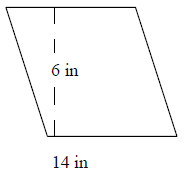### Explanation

Step 1:

Area of Parallelogram = b × h; b = base = 14 in; h = height = 6 in.

Step 2:

Area of given parallelogram = 14 × 6 = 84 square in.

Q 2 - Find the area of the following parallelogram.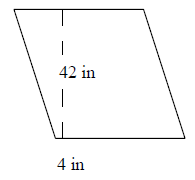### Explanation

Step 1:

Area of Parallelogram = b × h; b = base = 4 in; h = height = 42 in.

Step 2:

Area of given parallelogram = 4 × 42 = 168 square in.

Q 3 - Find the area of the following parallelogram.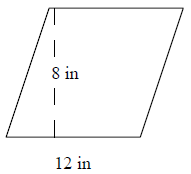### Explanation

Step 1:

Area of Parallelogram = b × h; b = base = 12 in; h = height = 8 in.

Step 2:

Area of given parallelogram = 12 × 8 = 96 square in.

Q 4 - Find the area of the following parallelogram.### Explanation

Step 1:

Area of Parallelogram = b × h; b = base = 9 in; h = height = 6 in.

Step 2:

Area of given parallelogram = 9 × 6 = 54 square in.

Q 5 - Find the area of the following parallelogram.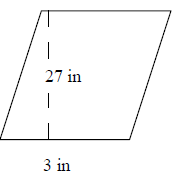### Explanation

Step 1:

Area of Parallelogram = b × h; b = base = 3 in; h = height = 27 in.

Step 2:

Area of given parallelogram = 3 × 27 = 81 square in.

Q 6 - Find the area of the following parallelogram.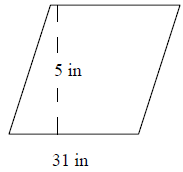### Explanation

Step 1:

Area of Parallelogram = b × h; b = base = 31 in; h = height = 5 in.

Step 2:

Area of given parallelogram = 31 × 5 = 155 square in.

Q 7 - Find the area of the following parallelogram.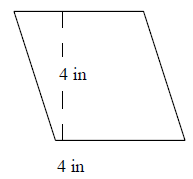### Explanation

Step 1:

Area of Parallelogram = b × h; b = base = 4 in; h = height = 4 in.

Step 2:

Area of given parallelogram = 4 × 4 = 16 square in.

Q 8 - Find the area of the following parallelogram.### Explanation

Step 1:

Area of Parallelogram = b × h; b = base = 8 in; h = height = 5 in.

Step 2:

Area of given parallelogram = 8 × 5 = 40 square in.

Q 9 - Find the area of the following parallelogram.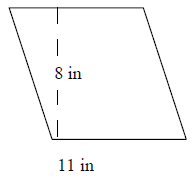### Explanation

Step 1:

Area of Parallelogram = b × h; b = base = 11 in; h = height = 8 in.

Step 2:

Area of given parallelogram = 11 × 8 = 88 square in.

Q 10 - Find the area of the following parallelogram.### Explanation

Step 1:

Area of Parallelogram = b × h; b = base = 40 in; h = height = 15 in.

Step 2:

Area of given parallelogram = 40 × 15 = 600 square in.

area_of_parallelogram.htm